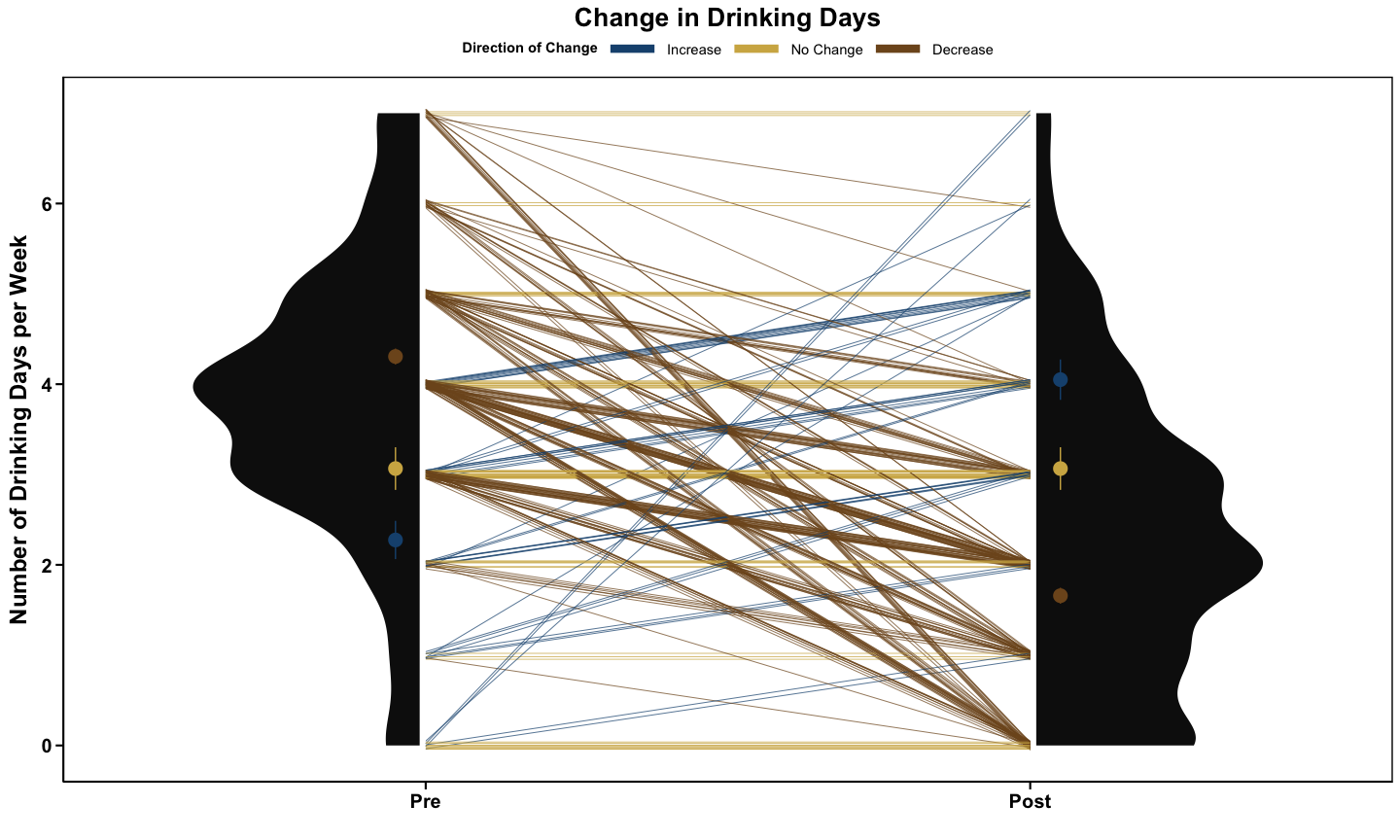# Introduction to Corset Plots

Corset plots are a visualization technique used to visualize repeat measures at 2 time points (such as pre- and post- data). Specifically, corset plots visualize the distribution of measurements at each time point, as well as the trajectories of individual change. This method of visualization is ideal for showing the heterogeneity of data and differences by sub-groups.

The ‘ggcorset’ package relies on ‘ggplot2’ to make its visualizations, allowing for easy customization and integration with other ‘ggplot2’ objects. This vignette will introduce uses of this visualization technique, as well as how to make customizations using ‘ggplot2’. For specific functionality, the GitHub README1 or the CRAN Package Manual2 should be consulted.

## Data Structure

The ‘ggcorset’ package provides two functions – `gg_corset()` and `gg_corset_elongated()` – which produce visualizations for wide and long data formats, respectively. The visualizations produced by these functions are identical.

The c_var variable is a user-defined variable that make the individual-level changes interpretable, and should be chosen strategically. This variable can be a discrete value reflecting change types or sub-groups. It can also be a continuous value to highlight levels of change. As such, various `c_var` strategies are outlined in this vignette.

## Anatomy of a Corset Plot

A corset plot consists of:

1. distribution at each time point using half violins (overall distributions and/ or distributions by group);

2. individual trajectories of change between each time point; and optionally

3. ‘eyelets’ showing either the standard error mean (SEM), or mean +/- 1 standard deviation (SD) by groupThe plot above was created using the sample ggcorset data “drinkdays”, and by creating a variable which captures the direction of change across the two time points. The `theme_ggcorset()` was used to polish the visualization alongside a few ggplot2 commands to customize the titles and axes, as denoted below:

``````library(ggcorset)
library(ggplot2)

# LOAD "drinkdays" data from the ggcorset package
data("drinkdays")

# Magnitude of change: Subtract T1 from T2
drinkdays\$change <- drinkdays\$time2-drinkdays\$time1

# Direction of Change
drinkdays\$direction <- ifelse(drinkdays\$change<0,"Decrease",
ifelse(drinkdays\$change>0,"Increase","No Change"))
drinkdays\$direction <- factor(drinkdays\$direction,
levels = c("Increase","No Change","Decrease"))

# CORSET PLOT: Basic plot + theme_ggcorset()
plot <- gg_corset(drinkdays, y_var1 = "time1", y_var2 = "time2",
c_var = "direction", group = "id", eyelets = T) +
theme_ggcorset()

plot +
# Changes legend title, and selects a colour-palette
scale_colour_manual("Direction of Change",
values = MetBrewer::met.brewer("Ingres",3)) +
# Changes the plot title
ggtitle("Change in Drinking Days") +
# Changes the y-axis title
ylab("Number of Drinking Days per Week") +
# Changes the x-axis title (removes in favour of the 2 time point labels below)
xlab("") +
# Changes the labels of the 2 time points (on the x-axis)
scale_x_discrete(labels = c("Pre","Post")) +
# Makes the legend lines thicker
guides(colour = guide_legend(override.aes = list(linewidth = 3)))``````

## Faceted Corset Plots

Corset plots can also be faceted using the `faceted = TRUE` argument. There are 3 design options available using the `facet_design`. Eyelets can be used in conjunction with the faceted corset plots, if desired. The default `facet_design` is “original” which simply facets the corset plots by the `c_var`.

The “group” option allows for the overall distribution to be plotted in the same colour as the `vio_fill` whilst a semi-opaque distribution of the `c_var` is situated ontop the overall distribution.

``````gg_corset(drinkdays, y_var1 = "time1", y_var2 = "time2",
c_var = "direction", group = "id", faceted = T, facet_design = "group") +
theme_ggcorset() +
scale_colour_manual("Direction of Change",
values = MetBrewer::met.brewer("Demuth",3, direction = -1))  +
scale_fill_manual(values = MetBrewer::met.brewer("Demuth",3, direction = -1))  +
ggtitle("Change in Drinking Days") +
ylab("Number of Drinking Days per Week") + xlab("") +
scale_x_discrete(labels = c("Pre","Post")) +
guides(colour = guide_legend(override.aes = list(linewidth = 3)))``````